SYLLABUS  Previous: 4.2 Linear equations.  Up: 4 FOURIER TRANSFORM  Next: 4.4 Non-linear equations.

4.3 Aliasing, filters and convolution.

Slide : [ Aliasing - Filters - Convolution || VIDEO login]

One of the beauties when using Fourier transforms, is the ability to work with a spectrum of modes and act on each of the components individually with a filter. By sampling the function over a period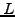with a finite number of values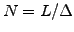, where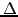is the size of the sampling interval, the spectrum gets truncated at the shortest wavelength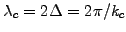called Nyquist critical wavelength: this corresponds to exactly 2 mesh points per wavelength, which does however not mean that shorter wavelengths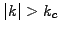do not contribute to the Fourier coefficients (4.1#eq.1). Figure 4.3#fig.1 illustrates how they get aliased back into the lower components of the spectrum.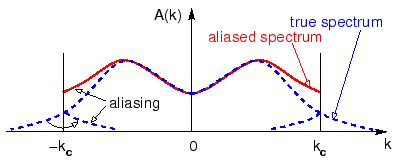This can be important for the digital data acquisition of an experiment, where the signal has to be low-pass filtered before it is digitally sampled. Figure 4.3 shows that even with the greatest precautions, such an aliasing can sometimes not be avoided, an needs then to be correctly interpreted.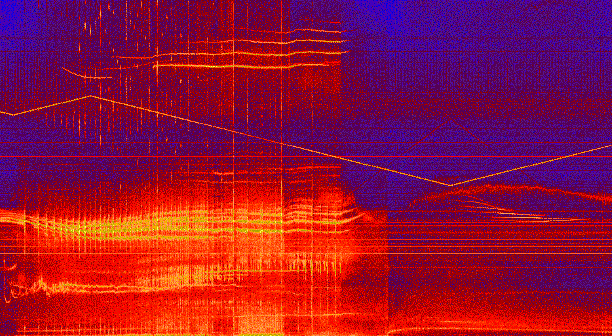It is easy to design a filter in Fourier space simply by multiplying the spectrum by a suitable filter function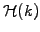(exercise 4.2). Simply remember that

• to keep the data real after transforming back to X-space, you must keep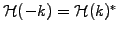, for example by choosing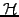real and even in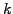,
• the filter has to be defined in the entire interval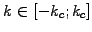and should be smooth to avoid phase errors and dampings for wavelengths that appear with sharp edges.

Although they are present right from the beginning when the initial condition is first discretized (try to initialize and propagate an aliased cosine with ICWavelength=1.05 mesh points per wavelength using the JBONE applet above), aliases do not actually interfere with the resolution of linear equations. The story is however different for spatial non-linearities such as the quadratic term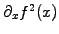that is responsible for the wave-breaking (1.3.4#eq.1). Indeed, think of the convolution theorem, which tells that the Fourier transform of a convolution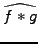is just the product of the individual Fourier transforms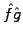. The converse is unfortunately also true: what can be viewed as a simple product in X-space becomes a convolution in K-space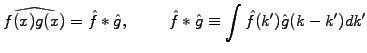(1)

or in discrete form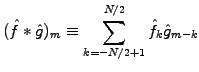(2)

For the quadratic wave-breaking non-linearity, this shows that a short wavelength component such as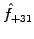in a sampling with 64 points, will incorrectly pollute'' a long wavelength channel through aliasing: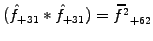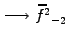. A simple cure for this, is to expand the size of the arrays by a factor two before the convolution takes place and pad them with zeros; changing the representation to calculate the multiplication of arrays twice the original size, the upper part of the spectrum is then simply discarded after the data has been transformed back. The entire procedure is illustrated in the coming section, where the non-linear Korteweg-DeVries (1.3.4#eq.3) and Burger equations (1.3.4#eq.2) are solved with a convolution in Fourier space.

SYLLABUS  Previous: 4.2 Linear equations.  Up: 4 FOURIER TRANSFORM  Next: 4.4 Non-linear equations.